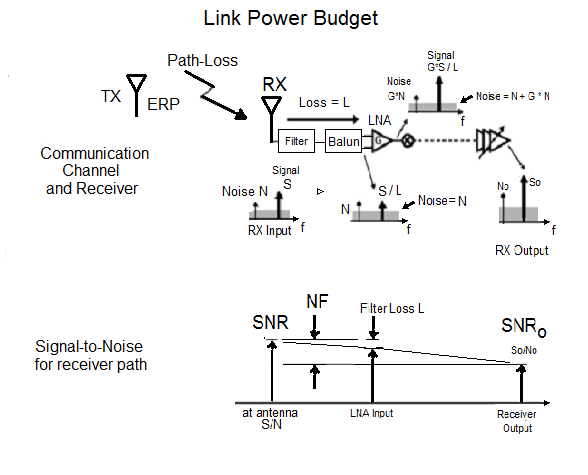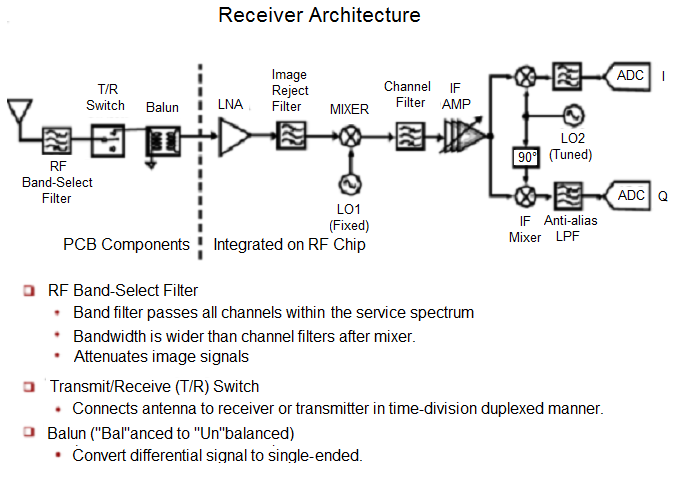## Tools for Estimating Range of RF Communication

Marcel Merchat
RF and Microwave Engineer

### The key concepts of formulating a power link budget include these factors:

• Friis Transmission Formula for signal loss over RF Channel Distance
• Power Spectrum Density
• Thermal Noise
• Signal-to-Noise Ratio
• Quantization Noise
• Bit Error Rate
• Shannon's Theorem for Channel CapacityERP: Effective Radiated Power ERP: Effective Radiated Power Path-Loss: Loss in RF Channel SNR: Signal-to-Noise (S/N) at Antenna N: Noise Floor at Antenna NF: Receiver noise figure SNRo: S/N at receiver output

The signal-to-noise ratio of the radiated signal is very high when there is no external interference. The signal to noise ratio at the receiver input is much lower because of thermal noise generated inside the receiver.

## Maximum Transmitter Power

#### In some cases, government regulations limit transmit power directly and in other cases there is a limit for power spectral density. As an example, consider the WLAN 802.11a limit of 2.5-mW per MHz over a 16-Mhz bandwidth. The maximum effective transitted power is 40 mW or 16 dBm.

BW <- 16                ## Bandwidth is 16 MHz
PSD <- 0.0025           ## PSD is 2.5 mW/MHz
ERP <- 1000 * BW * PSD  ## Total power for 16-MHz band

##  "The maximum effective radiated power for the transmitter is  40 mW."

##  "This is equivalent to  16.0 dBm."


## RF Path Loss

#### To cover 300 feet at 5-GHz requires a path loss of 86 dB. We describe this important antenna problem separately in another slide show. This results in a receiver signal of -70 dBM. The 802.11a specification requires that the signal must not exceed -65 dBm at 300 feet.

pathlossdB <- 86
receiver_signal <- ERPdB - pathlossdB ## Signal at 300 feet

##  "The signal at the receiver is  -70.0 dBm."


## Thermal Noise Floor

#### The noise floor at the receiver input is -174 dBm/Hz over the 16 MHz bandwidth. This generated noise power of -102 dBm at the input ot the receiver.

thermaln <- -174         ## PSD/Hz of thermal noise in dBm units
p <- 10^(thermaln/10)    ## convert to real number
pbw <- p * 16 * 10^6     ## Multiply by 16 MHz
floor <- 10 * log10(pbw) ## Convert to dBm
num <- formatC(floor, format = "f", digits = 1)

##  "The noise level at the antenna is  -102.0 dBm."## Required Bit Error Rate for Receiver

#### For 64 QAM, the required signal-to-noise is 27 dB at 54 MHz. This is an interesting problem in itself and will be covered by a separate slides. Since the packet length for 802.11a is 8-kB and assume error-correction-coding can handle 10% data loss, our BER requirement is approximately $10^{-5}$ as follows:

##### $(1-BER)^{8000}=1-0.1$.
BER <- 1 -  10^(log10(0.9)/8000)

##  "The required bit error rate (BER) is  0.000013"


## Required Noise Figure for Receiver

### NFR = 16 dBm - 86 dBm - 27 dBm - (-102 dBm) = 5 dBm

#### The noise figure of the receiver (NFR) must be 5 dB or lower.

##  "The required signal-to-noise ration for the receiver is  4.98 dB"


August 2, 2017